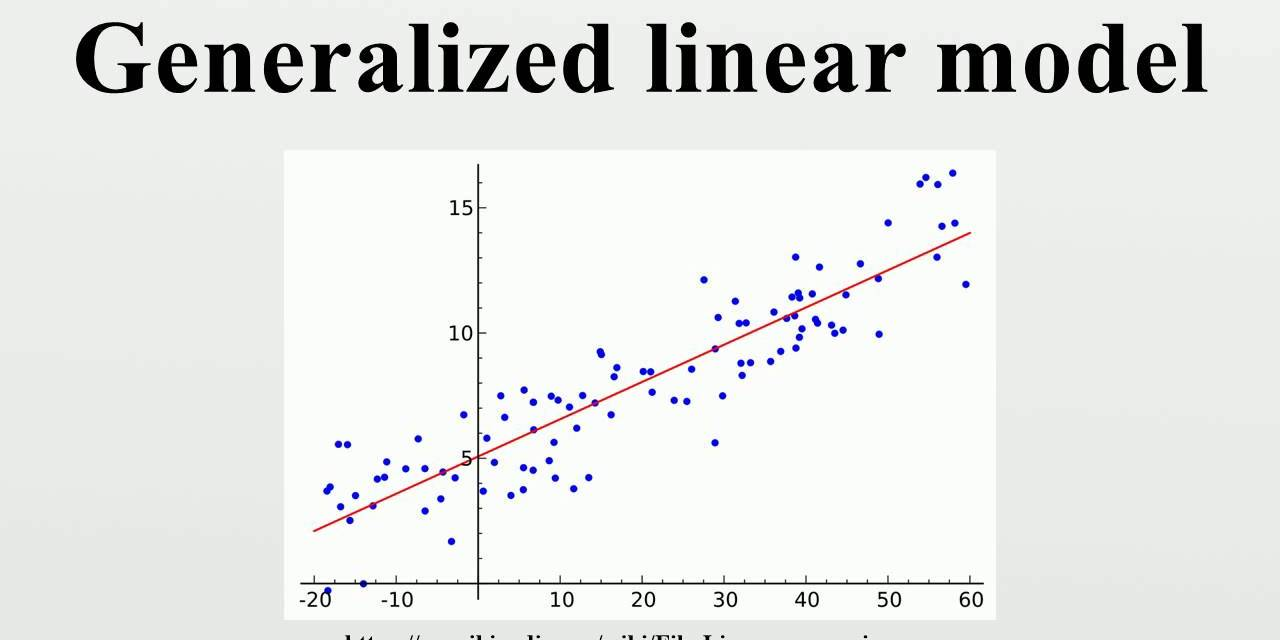## 线性模型

$$y = mx + b$$

$$成功 = \alpha * 实力 + （1 - \alpha）* 运气$$

## 大系数和新常态

$$y = \beta_0 + \beta_1 x_1 + \cdots + \beta_px_p + \epsilon$$